Tamilnadu State Board New Syllabus Samacheer Kalvi 12th Business Maths Guide Pdf Chapter 6 Random Variable and Mathematical Expectation Ex 6.1 Text Book Back Questions and Answers, Notes.

## Tamilnadu Samacheer Kalvi 12th Business Maths Solutions Chapter 6 Random Variable and Mathematical Expectation Ex 6.1

Question 1.
Construct cumulative distribution function for the given probability distribution.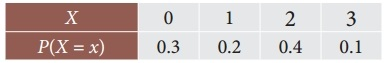Solution:
F(0) = P(x ≤ 0) = p(0) = 0.3
F(1) = P(x ≤ 1) = p(0) + p(1)
= 0.3 + 0.2 = 0.5
F(2) = P(x ≤ 2) = P(0) + P(1) + P(2)
= 0.3 + 0.2 + 0.4 = 0.9
F(3) = P(x ≤ 3) = P(0) + P(2) + P(3) + P(4)
= 0.3 + 0.2 + 0.4 + 0.1 = 1Question 2.
Let X be a discrete random variable with the following p.m.f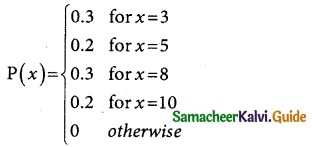Find and plot the c.d.f. of X.
Solution:
F(3) = P(x ≤ 3) = P(3) = 0.3
F(5) = P(x ≤ 5) = P(x = 3) + (x = 5)
= 0.3 + 0.2 = 0.5
F(8) = P(x ≤ 8) = P(3) + P(5) + P(8)
= 0.3 + 0.2 + 0.3
= 0.8
F(10) = P(x ≤ 10)
= P(3) + P(5) + P(8) + P(10)
= 0.3 + 0.2 + 0.3 + 0.2
= 1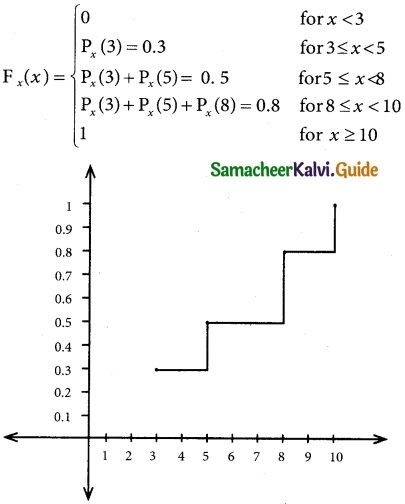Question 3.
The discrete random variable X has the following probability function.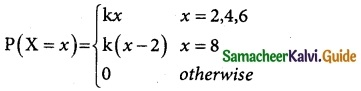where k is a constant. Show that k = $$\frac { 1 }{18}$$
Solution:
From the data
P(x = 2) = kx = 2k
P(x = 4) = kx = 4k
P(x = 6) = kx = 6k
P(x = 8) = k(x – 2)
= k(8 – 2)
= 6k
Since P(X = x) is a probability mass function
$$\sum_{x=2}^{8}$$ P(X = x) = 1
$$\sum_{i=2}^{∞}$$ P(xi) = 1
(i.e) P(x = 2) + P(x = 4) + P(x = 6) + P(x = 8) = 1
2k + 4k + 6k + 6k = 1
18k = 1
∴ k = $$\frac { 1 }{18}$$Question 4.
The discrete random variable X has the probability functionSolution:
$$\sum_{i=1}^{\infty} p\left(x_{i}\right)=1$$
gives k + 2k + 3k + 4k = 1
⇒ 10k = 1
⇒ k = $$\frac{1}{10}$$ = 0.1

Question 5.
Two coins are tossed simultaneously. Getting a head is termed a success. Find the t probability distribution of the number of successes.
Solution:
Let X is the random variable which counts the Number of Heads when the coins are tossed the outcomes are stated belowQuestion 6.
The discrete random variable X has the probability function.(i) Find k
(ii) Evaluate p( x < 6), p(x ≥ 6)and p(0 < x < 5) (iii) If P(X ≤ x) > 1, 2 then find the minimum value of x.
Solution:
(i) Since the condition of probability mass function
$$\sum_{i=1}^{\infty} p\left(x_{i}\right)=1$$
$$\sum_{i=0}^{7} p\left(x_{i}\right)=1$$
0 + k + 2k + 2k + 3k + k2 + 2k2 + 7k2 + k = 1
⇒ 10k2 + 9k – 1 = 0
⇒ (10k – 1) (k + 1) = 0
⇒ k = $$\frac{1}{10}$$ and k = -1
Since p(x) cannot be negative, k = -1 is not applicable. Hence k = $$\frac{1}{10}$$(ii) P(x < 6)
P(x < 6) = P(x = 0) + P(x = 1) + P(x = 2)+ P(x = 3) + p(x = 4) + P(x = 5)
= 0 + k + 2k +2k + 3k + k²
= 8k + k²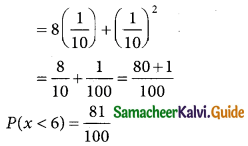P(x ≥ 6) = P(x = 6) + p(x = 7)
= 2k² + 7k² + k
= 9k² + kP(0 < x < 5)
= P(x = 1) + P(x = 2) + p(x = 3) + P(x = 4)
= k + 2k + 2k + 3k
= 8k = 8($$\frac { 1 }{10}$$)
∴ P(0 < x < 5) = $$\frac { 8 }{10}$$

(iii) We want the minimum value of x for which P(X ≥ x) > $$\frac { 1 }{2}$$
Now P(X ≤ 0) = 0 < $$\frac { 1 }{2}$$
P(X ≤ 1) = P(x = 0) + P(X = 1) = 0 + k = k
$$\frac { 1 }{10}$$ < $$\frac { 1 }{2}$$
P( X ≤ 2) = P(X = 0) + P(X = 1) + P(X = 2)
= 0 + k + 2k = 3k
$$\frac { 3 }{10}$$ $$\frac { 1 }{2}$$
P(X ≤ 3) = P(X = 0) + P(X = 1) + P(X = 2) + P(X = 2) + P(X = 3)
= 0 + k + 2k + 2k = 5k
= $$\frac { 5 }{10}$$ = $$\frac { 1 }{2}$$
P(X ≤ 4) = P(X = 0) + P(X = 1) + P(X = 2) + P(X = 2) + P(X = 3) + P(X = 4)
= 0 + k + 2k + 2k + 3k = 8k
= $$\frac { 8 }{10}$$ > $$\frac { 1 }{2}$$
This Shows that the minimum value of X for which P(X ≤ x) > $$\frac { 1 }{2}$$ is 4

Question 7.
The distribution of a continuous random variable X in range (-3, 3) is given by p.d.f.Verify that the area under the curve is unity.
Solution:
Since(-3, 3) in the range of given p.d.f
Area under the curve A = $$\int_{-∞}^{∞}$$ f(x)dx = $$\int_{-3}^{3}$$ f(x)dxHence the Area under the curve is unityQuestion 8.
A continuous random variable X has the following distribution function:Find (i) k and (ii) the probability density function.
Solution:
We have $$\frac{d}{d x}$$F(x) = f(x) ≥ 0, where F(x) is the distribution function and f(x) is the probability density function.
Here F(x) = 0 for x ≤ 1
f(x) = 0 for x ≤ 1
Again F(x) = 1 for x > 3
f(x) = $$\frac{d}{d x}$$ (1) = 0 for x > 3
In 1 < x ≤ 3, F(x) = k(x – 1)4
f(x) = $$\frac{d}{d x}$$ (k(x – 1)4) = 4k(x – 1)3
(i) We know that $$\int_{-\infty}^{\infty} f(x) d x$$ = 1
This gives $$\int_{1}^{3} 4 k(x-1)^{3} d x=1$$
$$\left[k(x-1)^{4}\right]_{1}^{3}=1$$
k[16 – 0] = 1
k = $$\frac{1}{16}$$
(ii) The probability density function is
f(x) = $$\frac{1}{4}(x-1)^{3}$$, 1 < x ≤ 3
= 0, otherwise

Question 9.
The length of time (in minutes) that a certain person speaks on the telephone is found to be a random phenomenon, with a probability function specified by the probability density function f(x) as(a) Find the value of A that makes f (x) a p.d.f.
(b) What is the probability that the number of minutes that person will talk over the phone is
(i) more than 10 minutes
(ii) less than 5 minutes, and
(iii) between 5 and 10 minutes.
Solution:
(a) Since f(x) is a probability density Function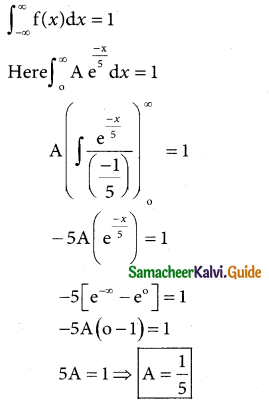b (i) more than 10 minutes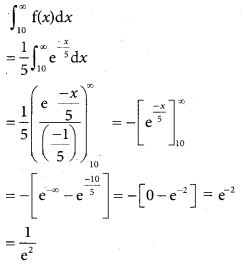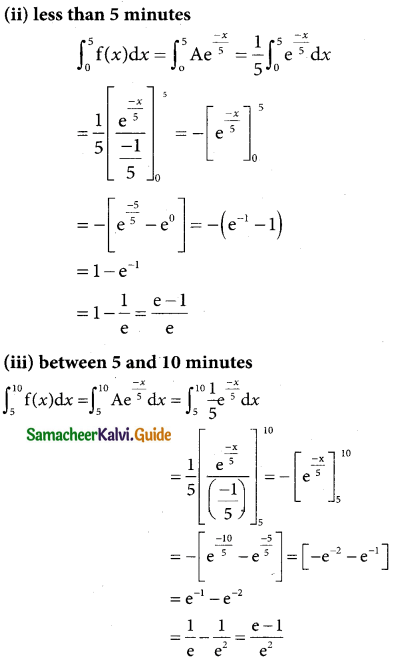Question 10.
Suppose that the time in minutes that a person has to wait at a certain station for a train is found to be a random phenomenon with a probability function specified by the distribution function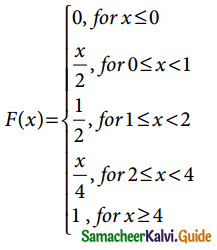(a) Is the distribution function continuous? If so, give its probability density function?
(b) What is the probability that a person will have to wait (i) more than 3 minutes, (ii) less than 3 minutes, and (iii) between 1 and 3 minutes?
Solution:
(i) Yes, the distribution function is continuous on [0, 4]
The probability density function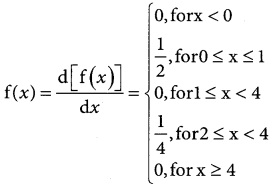(b) The probability that a person will have to wait
(i) more than 3 minutes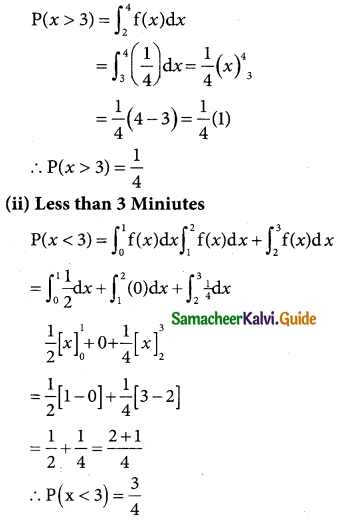(iii) between 1 and 3 minutesQuestion 11.
Define random variable.
Solution:
A Random variable is a set of possible values from a random experiment. The set of possible values is called the sample space. A random variable (r.v) is denoted by a capital letter such as X, Y and Z etc. If X and Y are r.v’s then X + Y is also an r.v.

Question 12.
Explain What are the types of random variables.
Solution:
Random variables are classified into two types namely discrete and continuous random variables these are important for practical applications in the field of Mathematics and Statistics.

Question 13.
Define discrete Random Variable
Solution:
A variable which can assume a finite number of possible values or an infinite sequence of countable real numbers is called a discrete random variable.
Examples:

1. Marks obtained in an exam.
2. The number of chocolates in a box.
3. The number of phone calls during a day.
4. The number of TV sets sold during a month by a dealer.

Question 14.
What do you understand by continuous random variables?
Solution:
A random variable X which can take on any value (integral as well as fraction) in the interval is called a continuous random variable.

Question 15.
Describe what is meant by a random variable
Solution:
A Random variable is a set of possible values from a random experiment. The set of possible values is called the sample space. A random variable (r.v) is denoted by a capital letter such as X, Y, and Z, etc. If X and Y are r.v’s then X + Y is also an r.v.Question 16.
Distinguish between a discrete and continuous random variable
Solution:Question 17.
Explain the distribution function of a random variable.
Solution:
The discrete cumulative distribution function or distribution function of a real-valued discrete random variable X takes the countable number of points x1, x2, …. with corresponding probabilities p(x1), p(x2),… and then the cumulative distribution function is defined by
Fx(x) = P(X ≤ x), for all x ∈ R
i.e. Fx (x) = $$\sum_{x \leq x}$$ p(xi)

Question 18.
Explain the terms (i) probability Mass function (ii) probability density function and (iii) probability
Solution:
(i) If X is a discrete random variable with distinct values x1, x2, …. xn, …, then the function, denoted by Px(x) and defined by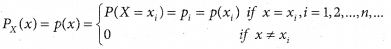This is defined to be the probability mass function or discrete probability function of X.

(ii) The probability that a random variable X takes a value in the interval [t1, t2] (open or closed) is given by the integral of a function called the probability density function fx(x):
P(t1 ≤ X ≤ t2) = $$\int_{t_{1}}^{t_{2}}$$ fx(x)dx.

(iii)The probability distribution of a random variable X is defined only when we have the various values oft the various values of the random variable e.g. x1, x2 …… xn togather with respective probabilities p1, p2, p3 …… p4 satisfying
$$\sum_{i=1}^{n}$$ Pi = 1Question 19.
What are the properties of
(i) discrete random variable and
(ii) Continuous random variable
Solution:
Discrete Random Variable:

• A variable which can take only certain values.
• The value of the variables can increase incomplete numbers
• Binomial, Poisson, Hypergeometric probability distributions come under this category
• Example: Number of students who opt for commerce in class 11, say 30, 35, 40, 45, and 50.

Continuous random variable:

• A variable which can take any value in a particular limit.
• Its value increases infractions but not in jumps.
• Normal, student’s t and chi-square distribution come under this category.
• Example: Height, Weight, and age of family members: 50.5 kg, 30 kg, 42.8 kg, and 18.6 kg.

Question 20.
State the properties of the distribution function.
Solution:

• Property 1: The distribution function F is increasing, (i.e) if x ≤ y, then F(x) ≤ F(y)
• Property 2: F(x) is continuous from right, (i.e) for each x ∈ R, F (x+) = F (x)
• Property 3: F (∞) = 1
• Property 4: F (-∞) = 0
• Property 5: F'(x) = f(x)
• Property 6: P(a ≤ X ≤ b) = F(b) – F(a)